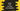# 2 different Kotlin programs to find the root of quadratic equation## How to find the roots of a quadratic equation in Kotlin:

In this post, we will learn how to find the roots of a quadratic equation in Kotlin with example. The program will take the values of the quadratic equation as inputs from the user and find the roots and print these out.

### How to find the quadratic equation roots:

A quadratic equation has the following form:

ax^2 + bx + c = 0

Where, a, b and c are real numbers and a is not equal to 0.

We can use the following formula to find the roots of this equation:

(-b ± √$b^2$ -4ac) / 2a

There are two roots, one is (-b + √$b^2$ -4ac) / 2a and (-b - √$b^2$ -4ac) / 2a

The $b^2$ -4ac part is called the determinant and its value determines wheather the roots will be real or imaginary. The determinant can be positive, negative or zero.

• The roots are real and different if the determinant is a positive value.
• The roots are real and equal if the determinant is equal to 0.
• The roots are imaginary if the determinant is negative.

The program will take the values of a, b and c as inputs from the user and print out the roots.

### Example 1: Kotlin program to find the roots of a quadratic equation by using if-else statements:

Below is the complete Kotlin program that finds the roots of a quadratic equation:

import kotlin.math.sqrt

fun main() {
println("Enter the values of a,b and c:")
val a = readLine()?.toDoubleOrNull()
val b = readLine()?.toDoubleOrNull()
val c = readLine()?.toDoubleOrNull()
val root1: String
val root2: String

if (a == null || b == null || c == null) {
println("Please enter valid inputs!!")
return
}

val determinant = (b * b) - 4 * a * c

if (determinant == 0.0) {
root1 = "%.2f".format(-b / (2 * a))
root2 = root1
} else if (determinant > 0) {
root1 = "%.2f".format((-b + sqrt(determinant)) / (2 * a))
root2 = "%.2f".format((-b - sqrt(determinant)) / (2 * a))
} else {
val real = -b / (2 * a)
val imaginary = sqrt(-determinant) / (2 * a)
root1 = "%.2f + %.2fi".format(real, imaginary)
root2 = "%.2f - %.2fi".format(real, imaginary)
}

println("First root: $root1\nSecond root:$root2")
}

In this program,

• It asks the user to enter the values of a, b and c. It reads the use input values and stores them in the variables a, b and c. We are using readLine to read the user input line and toDoubleOrNull method to convert it to a double value. For invalid inputs, it will return null.
• The first if statement checks if any of the values of a, b or c is null. If yes, it prints a message and returns from the method.
• We are calculating the determinant value and storing that value in the determinant variable.
• The if, else-if, else blocks checks the value of determinant and calculates the roots. The first root is stored in the root1 variable and the second root is stored in the root2 variable. Both are stored as string variables because we have to create these as strings if the value of determinant is less than zero.
• The last line is printing both of these roots.

It will print output as like below:

Enter the values of a,b and c:
20
10
11
First root: -0.25 + 0.70i
Second root: -0.25 - 0.70i

Enter the values of a,b and c:
x
77
12
Please enter valid inputs!!

### Example 2: Kotlin program to find the roots of a quadratic equation with when block:

We can also write the above program by using when block. It will give the same result:

import kotlin.math.sqrt

fun main() {
println("Enter the values of a,b and c:")
val a = readLine()?.toDoubleOrNull()
val b = readLine()?.toDoubleOrNull()
val c = readLine()?.toDoubleOrNull()
val root1: String
val root2: String

if (a == null || b == null || c == null) {
println("Please enter valid inputs!!")
return
}

val determinant = (b * b) - 4 * a * c

when {
determinant == 0.0 -> {
root1 = "%.2f".format(-b / (2 * a))
root2 = root1
}
determinant > 0 -> {
root1 = "%.2f".format((-b + sqrt(determinant)) / (2 * a))
root2 = "%.2f".format((-b - sqrt(determinant)) / (2 * a))
}
else -> {
val real = -b / (2 * a)
val imaginary = sqrt(-determinant) / (2 * a)
root1 = "%.2f + %.2fi".format(real, imaginary)
root2 = "%.2f - %.2fi".format(real, imaginary)
}
}

println("First root: $root1\nSecond root:$root2")
}

Output:

Enter the values of a,b and c:
3
4
11
First root: -0.67 + 1.80i
Second root: -0.67 - 1.80i

Enter the values of a,b and c:
12
0
12
First root: -0.00 + 1.00i
Second root: -0.00 - 1.00i